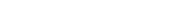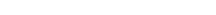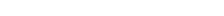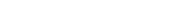xPoundalEncyclopedia
The poundal is a unit
Units of measurement
A unit of measurement is a definite magnitude of a physical quantity, defined and adopted by convention and/or by law, that is used as a standard for measurement of the same physical quantity. Any other value of the physical quantity can be expressed as a simple multiple of the unit of...

of force
Force
In physics, a force is any influence that causes an object to undergo a change in speed, a change in direction, or a change in shape. In other words, a force is that which can cause an object with mass to change its velocity , i.e., to accelerate, or which can cause a flexible object to deform...

that is part of the foot-pound-second system of units, in Imperial units introduced in 1877, and is from the specialized subsystem of English Absolut (a coherent system).The poundal is defined as the force necessary to accelerate 1 pound
Pound (mass)
The pound or pound-mass is a unit of mass used in the Imperial, United States customary and other systems of measurement...

to 1 foot per second, per second.
1 pdl = exactly.

## Background

English units require re-scaling of either force or mass to eliminate a numerical proportionality constant in the equation F = ma. The poundal represents one choice, which is to rescale units of force. Since a pound of force (pound force) accelerates a pound of mass (pound mass
Pound (mass)
The pound or pound-mass is a unit of mass used in the Imperial, United States customary and other systems of measurement...

) at 32.174 049ft/s2 (9.80665m/s2; the acceleration of gravity
Standard gravity
Standard gravity, or standard acceleration due to free fall, usually denoted by g0 or gn, is the nominal acceleration of an object in a vacuum near the surface of the Earth. It is defined as precisely , or about...

, g), we can scale down the unit of force to compensate, giving us one that accelerates 1 pound mass at 1ft/s2 rather than at 32.174 049ft/s2; and that is the poundal, which is approximately the pounds force.

For example, a force of 1200 poundals is required to accelerate a person of 150 pounds mass at 8 feet per second squared:The poundal-as-force, pound-as-mass system is contrasted with an alternate system in which pounds are used as force (pounds-force), and instead, the mass unit is rescaled by a factor of roughly 32. That is, one pound-force will accelerate one pound-mass at 32 feet per second squared; we can scale up the unit of mass to compensate, which will be accelerated by 1 ft/s2 (rather than 32 ft/s2) given the application of one pound force; this gives us a unit of mass called the slug, which is about 32 pounds mass. Using this system (slugs and pounds-force), the above expression could be expressed as:Note: Slugs (32.174 049) and poundals (1/32.174 049) are never used in the same system, since each exists to solve the same problem and will cancel each other out; both should not be used together.

Rather than changing either force or mass units, one may choose to express acceleration in units of the acceleration due to Earth's gravity
Standard gravity
Standard gravity, or standard acceleration due to free fall, usually denoted by g0 or gn, is the nominal acceleration of an object in a vacuum near the surface of the Earth. It is defined as precisely , or about...

(called g). In this case, we can keep both pounds-mass and pounds-force, such that applying one pound force to one pound mass accelerates it at one unit of acceleration (g):Expressions derived using poundals for force and for mass (or for force and slugs for mass) have the advantage of not being tied to conditions on the surface of the earth. Specifically, computing F = m·a on the moon or in deep space as poundals, ·ft/s2 or = slug·ft/s2, avoids the constant tied to acceleration of gravity on earth.